# SAT Math Multiple Choice Question 13: Answer and Explanation

### Test Information

Question: 13

3. If i =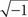, what is the product of (4 + 7i) and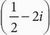?

• A. 16 -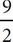i
• B. 14 +i
• C. 2 - 8i - 14i2
• D.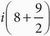A Use FOIL to multiply the two binomials together. The expression becomes 4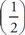- 8i + 7i- 14i2. Simplify the result by multiplying through where you can to get 2 - 8i +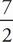i - 14i2. To combine the i terms, multiply 8 by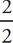to get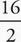. Now the expression is 2 -i +i - 14i2, which can be further simplified to 2 -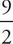i - 14i2. Substitute -1 for i2 and combine like terms: 2 -i + 14 = 16 -i, which is (A).